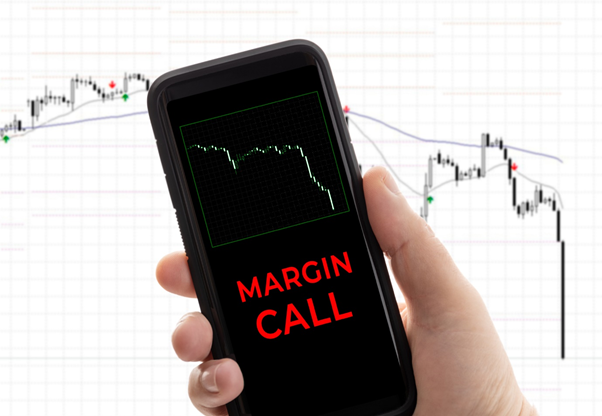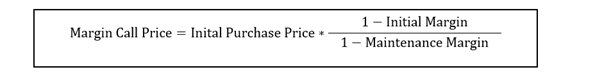# Margin Call

• Updated on

What is meant by the term margin call?

A margin call is a demand triggered by a lender when the value of the margin account falls below the level of the agreed margin requirement. The loan in a margin account has a periodic interest rate attached to it.

Summary
• A margin call is a demand triggered by a lender when the value of the margin account falls below the level of the agreed margin requirement.
• If a margin call is triggered, the borrower may add more cash or add more securities or clear off a part of the principal from the outstanding amount or place a sell order.## Image Source: © Avictorero | Megapixl.com

How is margin call price estimated?

The margin call price can be estimated using the following formula-In the above formula, initial purchase price is the price at which the investor bought the security; initial margin is the minimum percentage that the investor must pay; maintenance margin is the amount of equity that the lenders expect the borrower to maintain in the margin account.

What is meant by buying on margin?

Margin is the collateral that a borrower or investor deposits with the lender to cover against the risk of default. Buying on margin is an arrangement wherein investors buy securities by borrowing a part from the lender or broker. The marginable securities are used by the borrowers as collateral in their brokerage account.

Purchasing securities using margin is similar to buying more assets by using the existing cash (in the investor's hand) as collateral for the loan. For instance, if a broker has a margin requirement of 65% for the margin account and an investor B wants to buy securities worth \$1000, then the margin would be \$650, and B can borrow the rest from the broker.

What is a margin account?

We can understand the concept of a margin account with an example. Suppose X wants to buy stocks worth US\$ 50,000 in a margin account. X pays US\$ 15,000, and the broker lends the difference of US\$ 35000. X pays the interest on the loan amount, and the stocks are held as collateral.

When investors make investments using margin funds, if the value of the investments improves beyond the interest rate charged, then the investor’s returns will be better than if the investment was done in cash. In the above example the investor is set to gain on US\$15,000 he or she deploys in the trade while the asset purchased is worth US\$ 50,000. In other words the investor gets a leverage.

However, the downside is that if the market value of the securities declines, then the investor will be underwater on loan.

What triggers a margin call from the lender?

Depending on the market fluctuations, the prices of securities are bound to vary. Therefore, based on the current market price, if the value of a margin account falls below the account’s maintenance margin requirement.

Let us try to understand with the help of an example when a lender can trigger a margin call. Z wants to buy securities worth US\$ 200 with an initial margin of 60%, i.e. Z will use US\$ 120 as the initial margin and the balance US\$ 80 borrowed from a broker. If the maintenance margin is 30%, then the margin call price will be estimated using the above formula where

Initial purchase price= 200

Initial Margin= 60%

Maintenance Margin= 30%

So a margin call will be triggered if the price of the security declines below US\$ 114.28.

What is meant by excess margin deposit?

There is a minimum level that investors must maintain regarding the value of the collateral held in the margin account. When the deposit exceeds this minimum level, an excess margin deposit is said to exist.

Let us try to understand the situation of margin excess with the help of an example. Suppose investor Y wants to buy securities worth AU\$ 10,000. The brokerage firm mandates 20% maintenance requirement. So for the total investment of AU\$ 10,000, the investor has to maintain a margin of at least AU\$ 2,000. The investor has AU\$ 5,000 with him and intends to borrow the remaining AU\$ 5,000. The excess margin is the difference between the equity amount of the investor and the minimum margin requirement, i.e. AU\$ 3,000 (AU\$ 5,000 – AU\$ 2,000), in this case. The excess margin helps the lender cushion the impact of changes in the security price to some extent.

Suppose, if the value of the investment falls by 20%, the value of the securities will now be AU\$ 8,000. Since the borrowed amount is AU\$ 5,000, the residual amount, i.e. AU\$ 3,000, will be the value of the investor's equity. The margin requirement now is AU\$ 1,600 (20% of AU \$ 8,000). The excess margin is now AU\$ 1,400 (AU\$ 3,000 – AU\$ 1,600), compared to AU\$ 3,000, with some decline in the value of securities absorbed by the excess margin.

Now let us take another scenario where the value of the initial investment falls by 40%. The value of the securities will now be AU\$ 6,000. Since the borrowed amount is AU\$ 5,000, the residual amount, i.e. AU\$ 1,000, will be the value of the investor's equity. The margin requirement now is AU\$ 1,200 (20% of AU \$ 6,000). The margin requirement is higher than the equity invested, and hence, the investor will be asked to keep at least AU\$ 2,000 to maintain the margin requirements.

What can the borrower do if a margin call is triggered?

The margin call is triggered by the lender so that the margin account reverts to the excess margin position. The borrower can do this in the following ways-

• Add in more cash or
• Clear off a part of the principal from the outstanding amount or
• Place a sell orderImage Source: © Karpenkoilia | Megapixl.com

How can a margin call be avoided?

To avoid margin calls, instead of investing all their money, investors can set aside some amount as liquid cash deposits whose value will not change with market price fluctuations. Also, if a portfolio is diversified, there is a good chance that all the securities will not plummet together below the agreed maintenance margin. Assets with good trend should always be the preferred choice. Also, regular interest payments will keep a check on the outstanding amounts. In addition, as a proactive step, investors themselves can set a limit above the broker prescribed maintenance margin to top up the account on time so that a margin call is not triggered.

##### Top ASX Listed Companies

Definition:
We use cookies to ensure that we give you the best experience on our website. If you continue to use this site we will assume that you are happy with it. OK